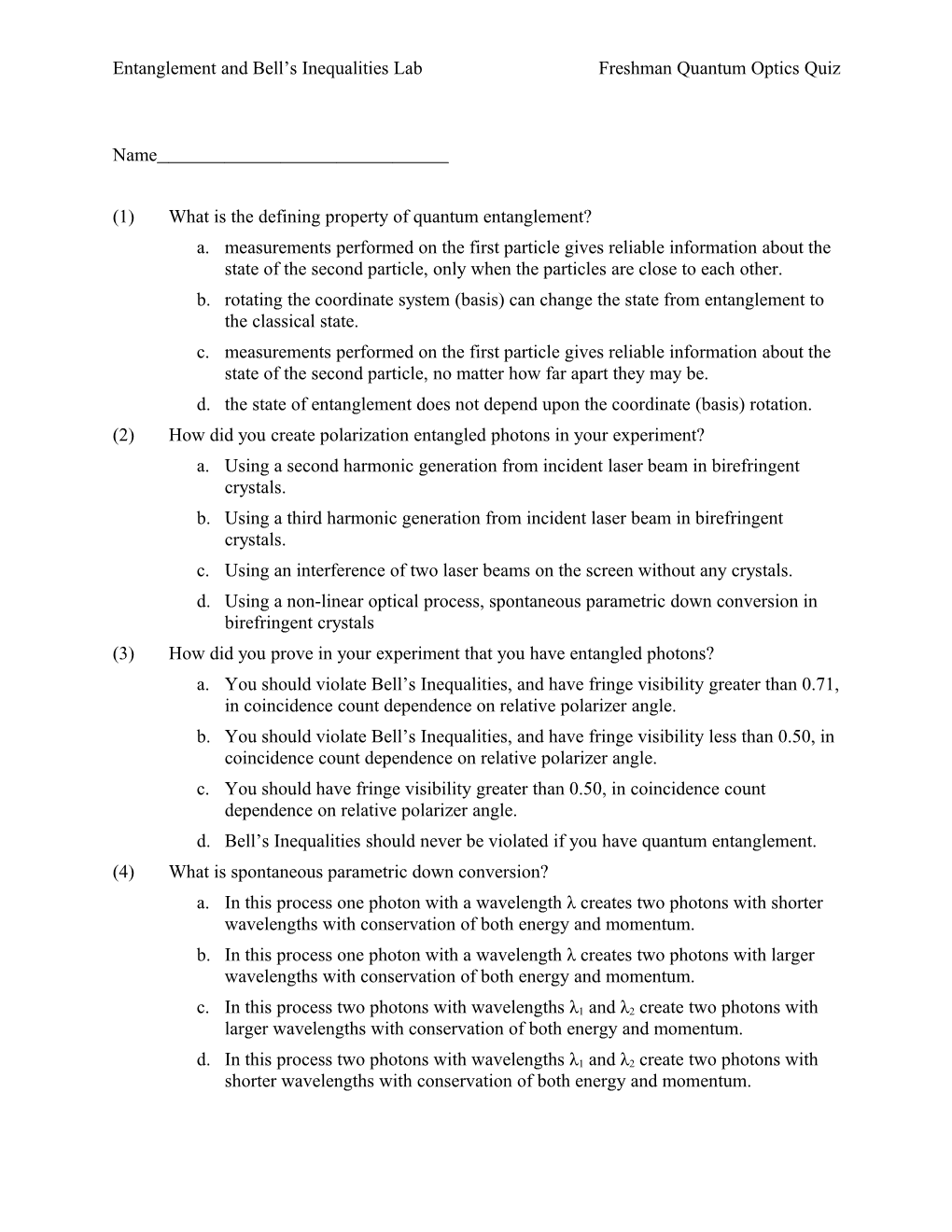# Entanglement and Bell S Inequalities Labfreshman Quantum Optics QuizEntanglement and Bell’s Inequalities LabFreshman Quantum Optics Quiz

1

Page #

Name

(1)What is the defining property of quantum entanglement?

1. measurements performed on the first particle gives reliable information about the state of the second particle, only when the particles are close to each other.
2. rotating the coordinate system (basis) can change the state from entanglement to the classical state.
3. measurements performed on the first particle gives reliable information about the state of the second particle, no matter how far apart they may be.
4. the state of entanglement does not depend upon the coordinate (basis) rotation.

(2)How did you create polarization entangled photons in your experiment?

1. Using a second harmonic generation from incident laser beam in birefringent crystals.
2. Using a third harmonic generation from incident laser beam in birefringent crystals.
3. Using an interference of two laser beams on the screen without any crystals.
4. Using a non-linear optical process, spontaneous parametric down conversion in birefringent crystals

(3)How did you prove in your experiment that you have entangled photons?

1. You should violate Bell’s Inequalities, and have fringe visibility greater than 0.71, in coincidence count dependence on relative polarizer angle.
2. You should violate Bell’s Inequalities, and have fringe visibility less than 0.50, in coincidence count dependence on relative polarizer angle.
3. You should have fringe visibility greater than 0.50, in coincidence count dependence on relative polarizer angle.
4. Bell’s Inequalities should never be violated if you have quantum entanglement.

(4)What is spontaneous parametric down conversion?

1. In this process one photon with a wavelength λ creates two photons with shorter wavelengths with conservation of both energy and momentum.
2. In this process one photon with a wavelength λ creates two photons with larger wavelengths with conservation of both energy and momentum.
3. In this process two photons with wavelengths λ1 and λ2 create two photons with larger wavelengths with conservation of both energy and momentum.
4. In this process two photons with wavelengths λ1 and λ2 create two photons with shorter wavelengths with conservation of both energy and momentum.

Questions #5-8: A sketch of your experimental set up is shown below. Please label items #5-8, using the following terms: one BBO crystal, two BBO crystals, detector, diode, interference filter, polarizer, quartz plate. (You may use terms more than once.)(5)______

(6)______

(7)______

(8)______

(9)For what purpose do you need a quartz plate?

1. To compensate for the phase between the vertically and the horizontally polarized components of the down converted photon as a result of different optical paths in the birefringent crystals.
2. To increase the phase difference between the vertically and the horizontally polarized components of the down converted photon as a result of different optical paths in the birefringent crystals.
3. To make the output photons from the BBO crystals horizontally polarized.
4. To make the output photons from the BBO crystals vertically polarized.

(10)What are Bell’s inequalities? Can you calculate them for some classical objects?

1. Classical relations which, in the case of entanglement, are violated for some angles of polarizers, they cannot be calculated in classical cases.
2. Bell’s Inequalities can be calculated only in the case of quantum mechanics.
3. Bell’s Inequalities are always violated in quantum mechanics (for all values of polarizer settings).
4. Classical relations which, in the case of entanglement, are violated for some angles of polarizers, they can be calculated in classical cases.

(11)How did you calculate them in your Lab?

1. We need to carry out 16 measurements of coincidence counts for definite polarizer angles at which the value of S has a maximum value.
2. We need to carry out 10 measurements of coincidence counts for definite polarizer angles at which the value of S has a minimum value.
3. We need to measure cos2 dependence of the coincidence count on the relative polarizer angle for 10 different settings with fringe visibility greater than 0.1.
4. We need to measure cos2 dependence of the coincidence count on the relative polarizer angle for 16 different settings with fringe visibility greater than 0.5.

(12)You know that you have the quantum state . How do you expect count B to change as you rotate polarizer B from 0˚ to 360˚, but polarizer A is set for a fixed angle ?

1. Single count B does change because the light is polarized.
2. Single count B depends upon the angle of polarizer A.
3. Single count B does not change because the light is not polarized.

(13)If polarizer A was removed how would you expect the coincidence count to change as you rotate polarizer B form 0˚ to 360˚?

1. Coincidence count changes as cos2 on the rotation angle of polarizer B.
2. Coincidence count does not change.

(14)What is the difference between coincidence plot with no polarizer A and coincidence plot with the polarizer A at =0?

1. In the case of polarizer A at α=0, the rotation of polarizer B changes the coincidence count as cos2 dependence on the relative polarizer angle.
2. There will be no difference in the coincidence plots.

(15)How do you define a fringe visibility?

1. (Imaximum+Iminimum)/(Imaximum-Iminimum)
2. (Imaximum-Iminimum)/(2Imaximum+Iminimum)
3. (Imaximum-Iminimum)/(Imaximum+Iminimum)

(16)What visibility should you have to violate Bell’s inequality?

1. Less than 0.71
2. Greater than 0.50
3. Greater than 0.71
4. Less than 0.50

(17)How does a count rate of APD detector A (singles’ count rate) depend on the angle  of a polarizer A and on the angle  of a polarizer B?

1. cos2(-)
2. does not depend upon  and 

(18)What are the conditions for maximum and minimum coincidence count rates for setup with two polarizers in front of each APD? (Please provide your answer in the space provided below.)